Science, Maths & Technology

### Become an OU student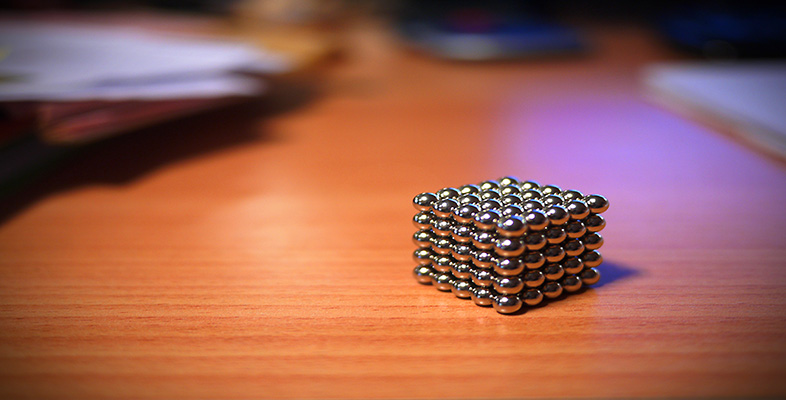Squares, roots and powers

Start this free course now. Just create an account and sign in. Enrol and complete the course for a free statement of participation or digital badge if available.

# 1.3 Square roots

Given any number, you now know how to find its square. But, given the squared number, how do you find the original number?

## Example 3

If the gardener in Example 1 had only 49 paving slabs, what size of square patio could she make?

You probably spotted that 49 is 7 × 7, or 72, so she could make a square patio 7 slabs by 7 slabs.

Since 72 = 49, 7 is the square root of 49, written

7 =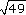.

Sometimes you can just look at a number and spot its square root, if the number is a ‘perfect square’ (i.e. the result of squaring a whole number). For example, 25 is a perfect square, and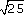= 5. But more often than not you will need to use your calculator for square roots, and it is important to be able to find rough estimates as a check on your calculator work. So if you wanted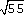, you would know that it would lie between= 7 and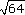= 8, and you would expect an answer of seven point something. (It is 7.416 …).

Technically, 7 is also a square root of 49, since (7)2 = 49. This is called the negative square root. The sign √ is customarily used to denote the positive square root, so= 7 and= 7.

In Example 3, only the positive square root is relevant (patios have positive length sides).

## Example 4

The owners of a new house, with a bare earth garden, see an advertisement for 44 square metres of turf, ‘free to a good home – only pay transportation’. They were planning a square lawn surrounded by flower beds.

Find a rough estimate for the square root of 44. Use your calculator to find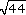and find the size of the square lawn which the turf would make.

Rounding 44 down to 40 doesn't help – you don't know the square root of 40 either! But you do know that 62 = 36 (which is less than 44) and 72 = 49 (which is greater than 44) solies between 6 and 7. You could leave the answer as ‘between 6 and 7’ or guess it as 6.5, say. The calculated answer is 6.6332 (rounded to four decimal places). So the turf would make a lawn about 6.6 m square.